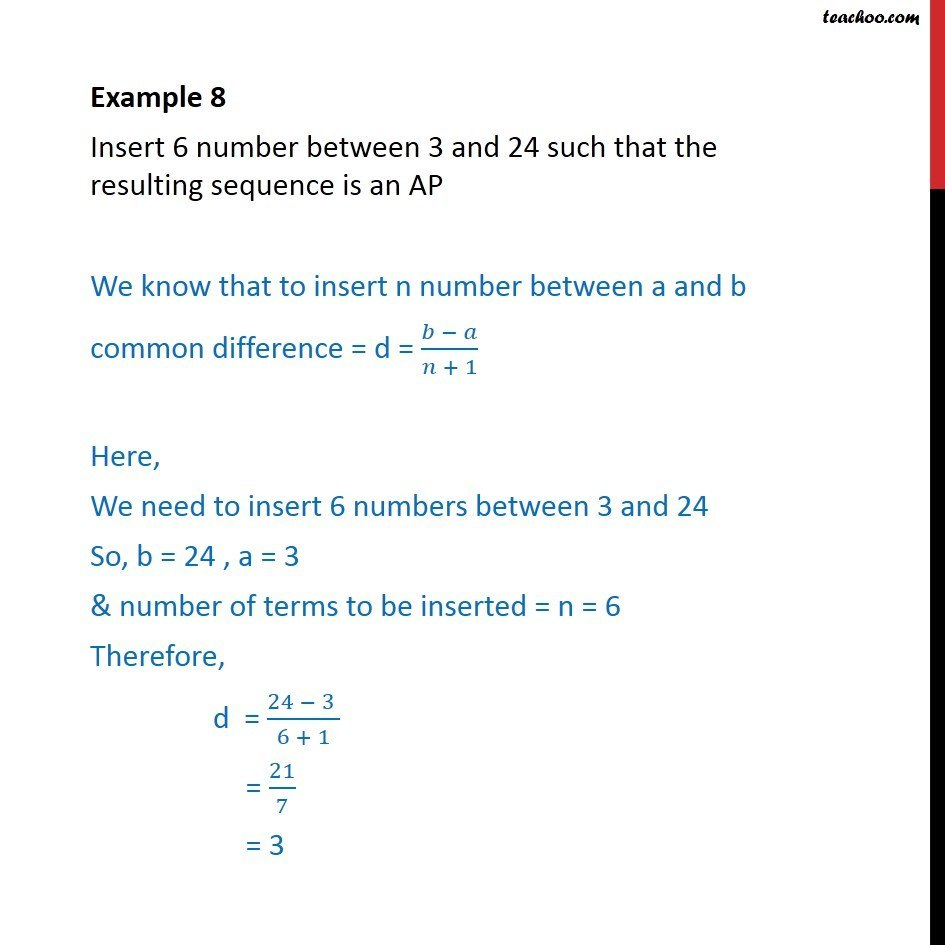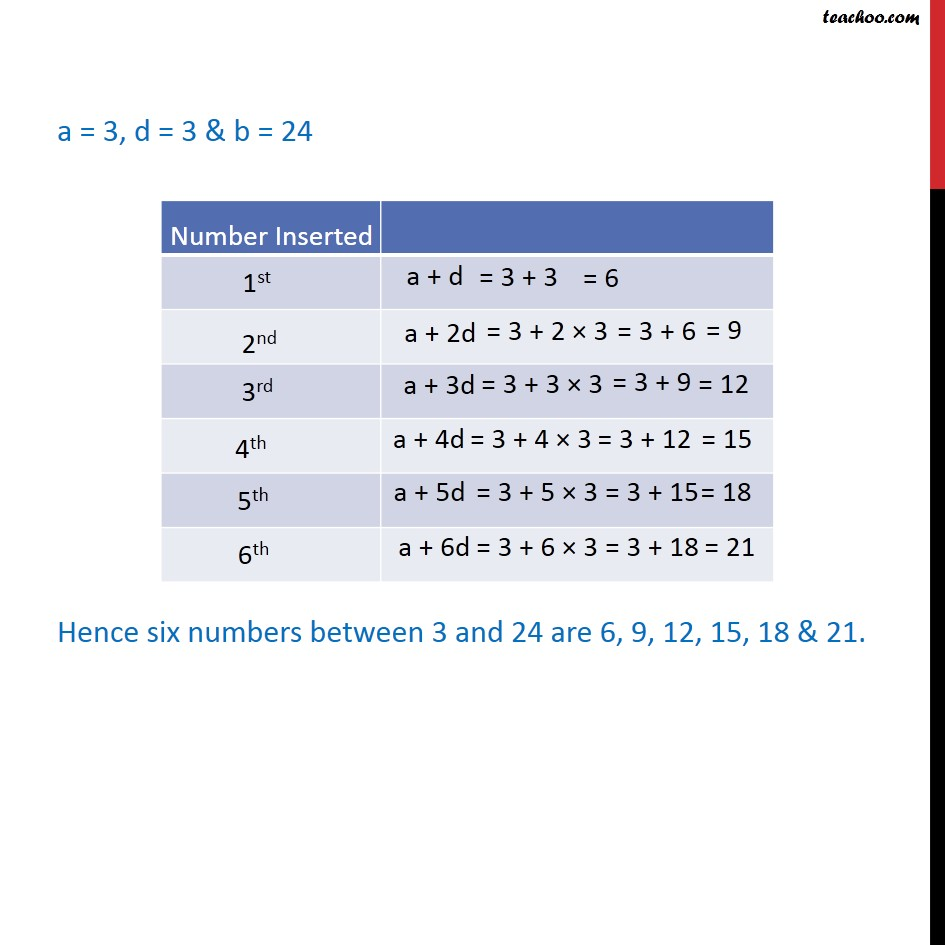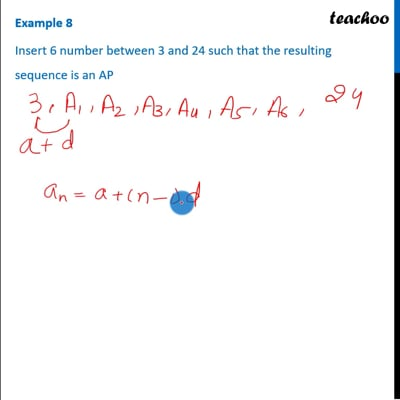Examples

Chapter 8 Class 11 Sequences and Series
Serial order wiseThis video is only available for Teachoo black usersThis video is only available for Teachoo black users

Learn in your speed, with individual attention - Teachoo Maths 1-on-1 Class

### Transcript

Question 5 Insert 6 number between 3 and 24 such that the resulting sequence is an AP We know that to insert n number between a and b common difference = d = (𝑏 − 𝑎)/(𝑛 + 1) Here, We need to insert 6 numbers between 3 and 24 So, b = 24 , a = 3 & number of terms to be inserted = n = 6 Therefore, d = (24 − 3 )/(6 + 1) = 21/7 = 3 a = 3, d = 3 & b = 24 Hence six numbers between 3 and 24 are 6, 9, 12, 15, 13 & 21.